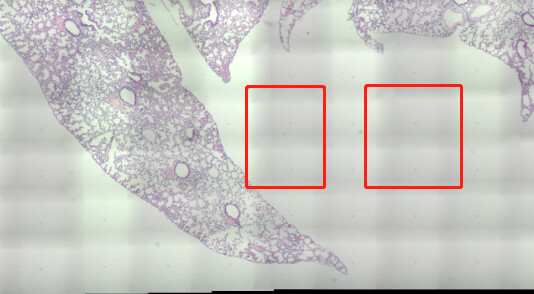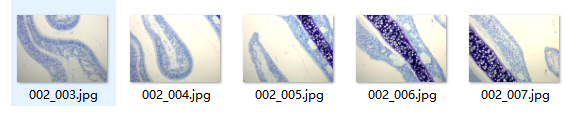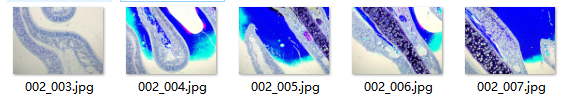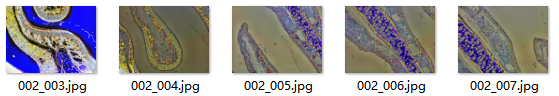# how to balance the illumination( Correction of Intensity Inhomogeneity) in itk Montage

hello, i use itk stitch microscope tiles to whole slide image, it works really good, yet there are some grid shadow in the blending area like thisi looked into it, it’s caused by the uneven illumination, this article mention that N4BiasFieldCorrectionImageFilter can fix this
according to N4BiasFieldCorrectionImageFilter doc
here is my code

``````import SimpleITK as sitk
import sys
import os

image = inputImage

maskImage = sitk.OtsuThreshold(inputImage, 0, 1, 200)
image = sitk.Shrink(inputImage,
[int(4)] * inputImage.GetDimension())
[int(4)] * inputImage.GetDimension())

corrector = sitk.N4BiasFieldCorrectionImageFilter()

numberFittingLevels = 4

corrector.SetMaximumNumberOfIterations([int(4)]
* numberFittingLevels)

log_bias_field = corrector.GetLogBiasFieldAsImage(inputImage)

corrected_image_full_resolution = inputImage / sitk.Exp( log_bias_field )
corrected_image_full_resolution = sitk.Cast(corrected_image_full_resolution, sitk.sitkUInt8)

output_file = "005_015_corrected.jpg"
sitk.WriteImage(corrected_image_full_resolution, output_file)
``````

the filter worked, yet the output image is gray, i want to cast the image to RGB, should i recompile SimpleITK to support cast the image to RGB? or is there any steps i was missing, it does not need a cast actually
here is my output image

Hello @allen,

The reason the image is gray is that when you read it you forced it to be gray by selecting the pixel type to be sitkFloat32.

The bias field correction works on a scalar (gray) image, so estimate it on the gray image as you’ve done and then apply to the color image (I’m not sure the bias field model holds in this case, but worth a try):

``````color_input_image = sitk.ReadImage(image_path)
bias_field = sitk.Exp( log_bias_field )
corrected_image_full_resolution = sitk.Compose([sitk.VectorIndexSelectionCast(color_input_image, i, sitk.sitkFloat32)/bias_field for i in range(3)])
corrected_image_full_resolution = sitk.Cast(corrected_image_full_resolution, sitk.sitkVectorUInt8)
output_file = "005_015_corrected.jpg"
sitk.WriteImage(corrected_image_full_resolution, output_file)
``````
2 Likes

The similar trick is used in the C++ example as well: compute bias field on the grayscale image, and apply it to color image.

1 Like

thanks zivy, the output image is RGB now, yet some of their color changed seriously.
the input imagesoutput imagesincrease the MaximumNumberOfIterations will makes it worseyet it still happen even if the MaximumNumberOfIterations is set to [1, 1, 1, 1]
i think it is caused by the bias_field, if the split bias_field removed, the compose return a normal image same as the input
is there anyway to fix this in N4BiasFieldCorrectionImageFilter
here is the code

``````def N4BiasFieldCorrection(image_path, output_file):
image = inputImage
maskImage = sitk.OtsuThreshold(inputImage, 0, 1, 200)
image = sitk.Shrink(inputImage,
[int(16)] * inputImage.GetDimension())
[int(16)] * inputImage.GetDimension())

corrector = sitk.N4BiasFieldCorrectionImageFilter()
corrector.SetMaximumNumberOfIterations([1, 1, 1, 1])
#     corrected_image = corrector.Execute(image)

log_bias_field = corrector.GetLogBiasFieldAsImage(inputImage)
bias_field = sitk.Exp(log_bias_field)
corrected_image_full_resolution = sitk.Compose([sitk.VectorIndexSelectionCast(color_input_image, i, sitk.sitkFloat32)/bias_field for i in range(3)])
corrected_image_full_resolution = sitk.Cast(corrected_image_full_resolution, sitk.sitkVectorUInt8)

sitk.WriteImage(corrected_image_full_resolution, output_file)
``````

sir, i wonder if there is a CompleteMontage.py or py version CorrectBias, i try to translate it

``````image = itk.imread(image_path)

region = image.GetLargestPossibleRegion()
bspliner = itk.BSplineControlPointImageFilter.New()
bspliner.SetInput(log_bias_field)
bspliner.SetSplineOrder(splineOrder)
bspliner.SetSize(region.GetSize())
bspliner.SetOrigin(image.GetOrigin())
bspliner.SetDirection(image.GetDirection())
bspliner.SetSpacing(image.GetSpacing())
bspliner.Update()
``````

its hard for me to fix the error, like itk does not have BSplineControlPointImageFilter attribute

Hello @allen,

When you read the color image the code is implicitly forcing it to be single channel:

``````inputImage = sitk.ReadImage(image_path, sitk.sitkFloat32)
``````

``````inputImage = sitk.ReadImage(image_path)
``````

Then convert it to grayscale based on your knowledge of the color representation. Then do the bias field correction on this grayscale image and apply it to the original three channel image.

For details on various color to grayscale conversion options see wikipedia. Code for converting from sRGB with gamma correction is available in this Jupyter notebook, function is called `srgb2gray`.

1 Like

This is rarely used, so it is no surprise to me that it is not wrapped. You could take a look at the wrappings of the other filters in that module, and add one for `BSplineControlPointImageFilter` yourself.

No, there isn’t. SimpleMontage.py is the only Python example we have for it.

Does C++ version work for you? I think it is easier to check that than write Python version of it. You need to point CMake at the examples’ CMakeLists to configure and compile it.

1 Like

hello @zivy sor for the idiot question, does “apply it to the original three channel image” means that apply the correction steps to all the 3 channels like below
I = (
I * sitk.Cast(I <= 0.0031308, sitk.sitkFloat32) * 12.92
+ I ** (1 / 2.4) * sitk.Cast(I > 0.0031308, sitk.sitkFloat32) * 1.055
- 0.055
)

``````def gamma_correction(I):
# nonlinear gamma correction
I = (
I * sitk.Cast(I <= 0.0031308, sitk.sitkFloat32) * 12.92
+ I ** (1 / 2.4) * sitk.Cast(I > 0.0031308, sitk.sitkFloat32) * 1.055
- 0.055
)
return sitk.Cast(sitk.RescaleIntensity(I), sitk.sitkUInt8)

channels = [
sitk.VectorIndexSelectionCast(color_input_image, i, sitk.sitkFloat32)
for i in range(color_input_image.GetNumberOfComponentsPerPixel())
]

res = [gamma_correction(c) for c in channels]
``````

and then compose 3 channel to rgb

``````corrected_image_full_resolution = sitk.Compose(res)
corrected_image_full_resolution = sitk.Cast(corrected_image_full_resolution, sitk.sitkVectorUInt8)
``````

this did not work to even the illumination

or it means that just replace the sitk.ReadImage(image_path, sitk.sitkFloat32) to srgb2gray result
like this below

``````def srgb2gray(image):
# Convert sRGB image to gray scale and rescale results to [0,255]
channels = [
sitk.VectorIndexSelectionCast(image, i, sitk.sitkFloat32)
for i in range(image.GetNumberOfComponentsPerPixel())
]
# linear mapping
I = 1 / 255.0 * (0.2126 * channels + 0.7152 * channels + 0.0722 * channels)
# nonlinear gamma correction
I = (
I * sitk.Cast(I <= 0.0031308, sitk.sitkFloat32) * 12.92
+ I ** (1 / 2.4) * sitk.Cast(I > 0.0031308, sitk.sitkFloat32) * 1.055
- 0.055
)
# i change sitkUInt8 to sitkFloat32 as the N4BiasFieldCorrectionImageFilter didn't support sitkUInt8
return sitk.Cast(sitk.RescaleIntensity(I), sitk.sitkFloat32)

def N4BiasFieldCorrection(image_path, output_file):
inputImage = srgb2gray(color_input_image)
image = inputImage
maskImage = sitk.OtsuThreshold(inputImage, 0, 1, 200)
image = sitk.Shrink(inputImage,
[int(16)] * inputImage.GetDimension())
[int(16)] * inputImage.GetDimension())

corrector = sitk.N4BiasFieldCorrectionImageFilter()
corrector.SetSplineOrder(3)
corrector.SetWienerFilterNoise(0.01)
corrector.SetBiasFieldFullWidthAtHalfMaximum(0.15)
corrector.SetConvergenceThreshold(0.00001)
corrector.SetMaximumNumberOfIterations([1, 1, 1, 1])

log_bias_field = corrector.GetLogBiasFieldAsImage(inputImage)
bias_field = sitk.Exp(log_bias_field)
corrected_image_full_resolution = sitk.Compose([sitk.VectorIndexSelectionCast(color_input_image, i, sitk.sitkFloat32)/bias_field for i in range(3)])
corrected_image_full_resolution = sitk.Cast(corrected_image_full_resolution, sitk.sitkVectorUInt8)

sitk.WriteImage(corrected_image_full_resolution, output_file)
``````

the color changed in its result too, and it does gamma correction twice i think

if both the 2 version above not what you mean, how to apply it to the original three channel image, i know rgb image can trans to hsv image, the v channel represent the intensity, but grey image only has one channel, how can i extract its intensity features and apply it to the original three channel

hello sir, i build CompleteMontage.cxx to exe file by cmake

here is its output

the left one is gen set doBiasCorrection doDenoising to false

``````CompleteMontage.exe E:\\projects\\ITKMontage\\examples\\SampleData_Balance E:\\projects\\ITKMontage\\examples\\output_balance montage.tiff 0 0
``````

right one is gen set doBiasCorrection doDenoising to true

``````CompleteMontage.exe E:\\projects\\ITKMontage\\examples\\SampleData_Balance E:\\projects\\ITKMontage\\examples\\output_balance montage.tiff
``````

the uneven intensity is much better, but the color changed(distortion?) in some tiles just like the sitk.N4BiasFieldCorrectionImageFilter result as upload above
logs: CompleteMontage.log (27.2 KB)

one of the intensity flat result

The total size of tiles is relatively large, i will upload the original data and config file if needed to reproduce

1 Like

At first I thought, since the tiles are large, the intensities might be overflowing the chosen types. But the accumulate types are big enough.:

Can you examine these files, and see whether it is the bias correction which corrupts/saturates the intensities:

If not, it must be invoked from here:

and the problem being in:

2 Likes

i would like to examine it sir, yet don’t know how.
it’s the same result if set doBiasCorrection to true and doDenoising to false, is this enough to make sure bias correction corrupts/saturates the intensities

left is only bias correction, right is bias correction and denoise
if its problem in itkTileMergeImageFilter, do you have any idea how to fix this

I meant look at the files named `baseFileName + "-flat" + fileNameExt` in the `outputPath`. Do these files have the problem, or is the problem present only in the final, stitched image?

the -flat file have a problem it self before stitch

1 Like

here is some original files can reproduce it

The problem is in the `CorrectBias` function:

Probably just a few formulas need to be adjusted there. I don’t have time to work on it this week (paper deadline March 1st). Lines you should pay attention to, and experiment with: 269, 297, 305 and 312.

1 Like

it’s all right. thanks for replying so many out of your busy schedule. I will try it tommorrowi tried to change the bias weight, it didn’t help. i found another way to balance the illumination by pyvips:

1、capture a white background with the camera slightly defocussed
2、scale pixels by 1/white

``````white = pyvips.Image.new_from_file(white_path)
vignette = (white.max() * 0.7 + white.min() * 0.3) / white

cur_frame = pyvips.Image.new_from_file(filename)
cur_frame = (cur_frame * vignette).cast("uchar")
``````

this method is really fast, less than 10ms per frame, and the result is acceptable for me
the test output

left is processed by adjust_gamma, its better than the original images but grid shadow are still obvious

``````def adjust_gamma(image, gamma=1.2):
# build a lookup table mapping the pixel values [0, 255] to
invGamma = 1.0 / gamma
table = np.array([((i / 255.0) ** invGamma) * 255 for i in np.arange(0, 256)]).astype("uint8")

# apply gamma correction using the lookup table
return cv2.LUT(image, table)
``````

right one is processed by pyvips vignette

thank you for all your help

1 Like

The generic inhomogeneity correction is a better-than-nothing cure when when other calibration methods are unavailable. Your solution seems good and fast.

1 Like